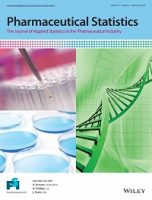# Open Access: A robust permutation test for the concordance correlation coefficient

Each week, we select a recently published Open Access article to feature. This week’s article comes from Pharmaceutical Statistics and develops a permutation test for the concordance correlation coefficient. The authors then use this test on real world examples related to cardic output measurements and endocardiographic imaging.The article’s abstract is given below, with the full article available to read here.

A robust permutation test for the concordance correlation coefficientPharmaceutical Statistics202120696– 709https://doi.org/10.1002/pst.2101

In this work, we developed a robust permutation test for the concordance correlation coefficient (ρc) for testing the general hypothesis H0 : ρc = ρc(0). The proposed test is based on an appropriately studentized statistic. Theoretically, the test is proven to be asymptotically valid in the general setting when two paired variables are uncorrelated but dependent. This desired property was demonstrated across a range of distributional assumptions and sample sizes in simulation studies, where the test exhibits robust type I error control in all settings tested, even when the sample size is small. We demonstrated the application of this test in two real world examples across cardiac output measurements and endocardiographic imaging.